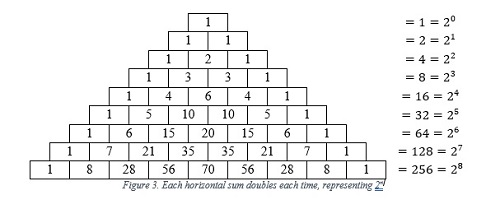﻿﻿﻿ The Golden Ratio | Centre of Foundation Studies for Agricultural Science
» ARTICLE » The Golden Ratio

The Golden Ratio

The golden ratio also known as “The Golden Section”, “Golden Mean” and “Divine Proportion”, is an irrational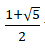number of  that gives a value of  and is often symbolized by the Greek letter  (phi). This ratio is considered a mathematical quantity that defines a beauty and wonder that can be found in nature.

The theory of this ratio arose when the Fibonacci numbers were introduced by Leonardo of Pisa, also known as Fibonacci, an Italian mathematician who lived during the 12th century. He is best known for popularizing the system of numbers that we use today.

The system of numbers starts from the problems related to breeding of rabbits. The problem involved simulating growth of a rabbit population over a series of months, assuming each pair of rabbits produces one new pair each month, and that it takes one month for a new pair to become reproductive. As Fibonacci solved the problem, he noticed that the population growth followed a particular sequence of numbers.

These numbers are known as the Fibonacci sequence, and it is obtained by adding the sum of the two preceding numbers in the sequence, for example , followed by , then  and so on. As Fibonacci continued to explore the mathematical properties of the Fibonacci sequence, he discovered that the ratio of each number to the previous number approached a constant value of approximately , which is now known as the golden ratio.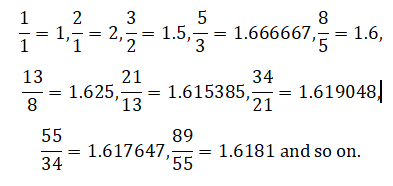This sequence is also known as the Fibonacci curve method.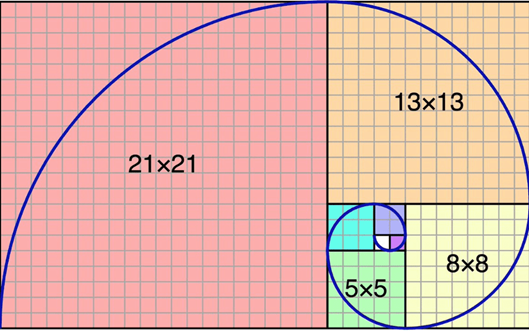Fibonacci curve method

The golden ratio was not actually discovered by Fibonacci, but rather it was observed and recorded by various mathematicians throughout history. However, Fibonacci did play a significant role in popularizing the concept and demonstrating its applications. The Fibonacci sequence is a mathematical marvel that has captured the imagination of mathematicians, scientists, and artists for centuries. Its appearance in nature and its connection to the Golden Ratio have led to its widespread use in many fields, and its applications continue to be discovered and explored. The curve of this golden ratio can be found in the growth of shells, the branching of trees and the formation of spiral galaxies. In many famous works of art and architecture, such as the Parthenon in Athens, Greece, and Leonardo da Vinci’s Mona Lisa, are believed to be based on the golden ratio.Golden ratio in Mona Lisa portrait.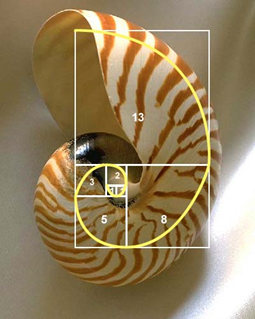Golden ratio in the growth of seashell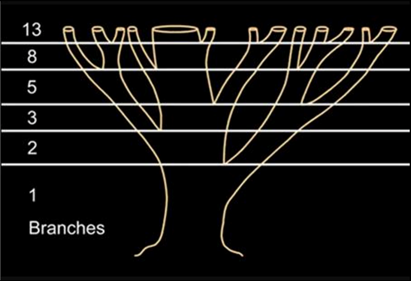Golden ratio in the branches of tree.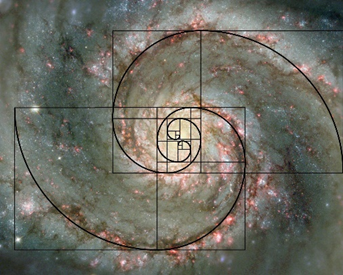Golden ratio in formation of galaxies.

By: Munirah binti Kamal, Mathematic Division, ASPer

Date of Input: 11/05/2023 | Updated: 11/05/2023 | emma

MEDIA SHARING